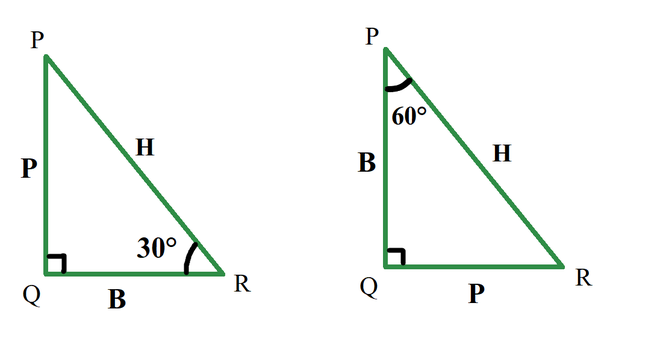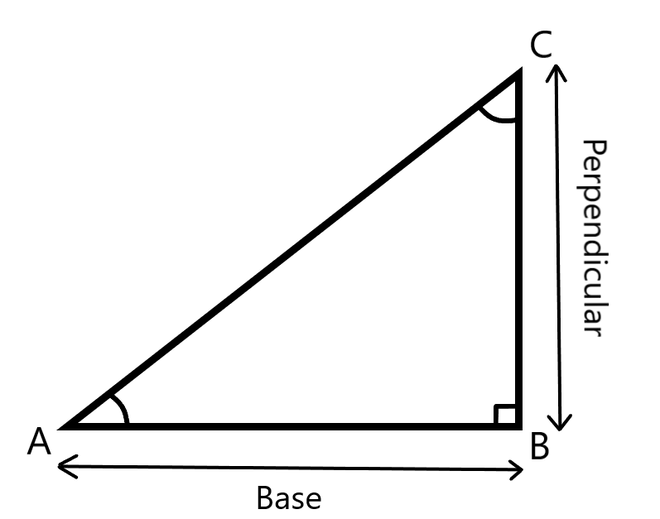# In a triangle ABC right angled at B, find the value of tan A

• Difficulty Level : Medium
• Last Updated : 12 Oct, 2021

Trigonometry is the branch of mathematics that deals with the relationship of sides with angles in a triangle. Trigonometry is based on the principle that “If two triangles have the same set of angles then their sides are in the same ratio”. Side length can be different but side ratios are the same. Trigonometry is very important in physics and mathematics, it is used to find, the height of towers, or distance between stars, and in GPS Navigation System.

### Trigonometric Functions

Trigonometric functions are also called circular functions or trigonometric ratios. The relationship of angles and sides is represented by these trigonometric functions. There are six trigonometric functions Sine, Cosine, Tangent, Cosecant, Secant, Cotangent. The sides representations for the six ratios are,

Attention reader! All those who say programming isn't for kids, just haven't met the right mentors yet. Join the  Demo Class for First Step to Coding Coursespecifically designed for students of class 8 to 12.

The students will get to learn more about the world of programming in these free classes which will definitely help them in making a wise career choice in the future.

• sin A = Perpendicular / Hypotenuse
• cos A = Base / Hypotenuse
• tan A = Perpendicular / Base
• cot A = Base / Perpendicular
• sec A = Hypotenuse / Base
• cosec A = Hypotenuse / Perpendicular.

Here, A is the angle opposite to the perpendicular side. Let’s learn what the Perpendicular, base, and hypotenuse of a right-angled triangle are,

1. Perpendicular: The side in front of the angle is perpendicular. In this case, the side in front of 30° is called it’s perpendicular.
2. Base: A base is one of the sides which touches angle, but hypotenuse can never be considered as base.
3. Hypotenuse: It is a side opposite to 90°. it is the largest side.

Note: Perpendicular and base changes as angle changes. In a triangle, a side is perpendicular for an angle, but the same side is a base for another angle, but the hypotenuse remains the same because it is a side opposite to angle 90°.As shown in the above diagram for the same triangle if considered angle 30° the perpendicular is the side PQ, but if considered angle 60° the perpendicular is side QR.

### In a triangle ABC right angled at B, find the value of tan A

Tan – The tan of an angle A is the ratio of lengths of perpendicular to the base.

Tan A = Perpendicular / Base

Let us see how a triangle ABC right angled at B looks like.Since Tan (A) = perpendicular / Base.

In a triangle right angled at B, Tan (A) = BC / AB.

### Sample Problems

Question 1: In a right angle triangle, angle A is 60°, and the base is 3m. Find the length of perpendicular.

Solution:

Given:  Base = 3m

Tan 60 = √3

P/B = √3

P/3 = √3/1

p = 3√3

Question 2: In a right angle triangle, angle A is 30°, and the Perpendicular is 3m. Find the length of the Base.

Solution:

Given:  perpendicular = 3m

Tan 30° = 1/√3

P/B = 1/√3

3/B = 1/√3

B = 3√3

Question 3: In a right angle triangle, for an angle A, the Perpendicular is 9√3m and the Base is 9m, find the angle A.

Solution:

Given:  perpendicular = 9√3m, Base = 9m.

Tan A = 9√3 / 9

Tan A = √3

Tan (60°) = √3

Angle A =  60°

My Personal Notes arrow_drop_up